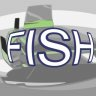# OBS LuaUnFish Lens 2022-05-21

This script adds the "Unfish/Fish Lens" video effect filter.
Compatible with OBS v21.1.2 and later.

Usage:
1. Download (or make it from the source code) the "filter-fish-lens.lua" script-file (MD5:B7094F27EEA1E2CE864BDB6A2E946ED2)
2. Navigate to main menu Tools>Scripts>Add_button("+") and select the file from the p1.
3. Right click over any obs video source and click Filters.
4. Under the section Effects Filters (right click menu, Add) will appear new filter named "UnFish/Fish Lens".
5. Enjoy!
Can be used to correct distortion of the fish-eye lenses of the video camera:Lua:
``````-- Inspired by Corner Pin effect filter v1.1 by khaver

obs = obslua
bit = require("bit")

TEXT_FILTER_NAME = 'UnFish/Fish Lens'
TEXT_FISH_POWER = 'Strength'

SETTING_FISH_POWER = 'fish_power'

source_def = {}
source_def.id = 'filter-fish-lens'
source_def.type = obs.OBS_SOURCE_TYPE_FILTER
source_def.output_flags = bit.bor(obs.OBS_SOURCE_VIDEO)

function set_render_size(filter)
target = obs.obs_filter_get_target(filter.context)

local width, height
if target == nil then
width = 0
height = 0
else
width = obs.obs_source_get_base_width(target)
height = obs.obs_source_get_base_height(target)
end

filter.width = width
filter.height = height
end

source_def.get_name = function()
return TEXT_FILTER_NAME
end

source_def.create = function(settings, source)
filter = {}
filter.params = {}
filter.context = source

set_render_size(filter)

obs.obs_enter_graphics()
if filter.effect ~= nil then
filter.params.fish_power = obs.gs_effect_get_param_by_name(filter.effect, 'fish_power')
filter.params.texture_width = obs.gs_effect_get_param_by_name(filter.effect, 'texture_width')
filter.params.texture_height = obs.gs_effect_get_param_by_name(filter.effect, 'texture_height')
end
obs.obs_leave_graphics()

if filter.effect == nil then
source_def.destroy(filter)
return nil
end

source_def.update(filter, settings)
return filter
end

source_def.destroy = function(filter)
if filter.effect ~= nil then
obs.obs_enter_graphics()
obs.gs_effect_destroy(filter.effect)
obs.obs_leave_graphics()
end
end

source_def.get_width = function(filter)
return filter.width
end

source_def.get_height = function(filter)
return filter.height
end

source_def.update = function(filter, settings)
filter.fish_power = obs.obs_data_get_double(settings, SETTING_FISH_POWER)

set_render_size(filter)
end

source_def.video_render = function(filter, effect)
if not obs.obs_source_process_filter_begin(filter.context, obs.GS_RGBA, obs.OBS_NO_DIRECT_RENDERING) then
return
end

obs.gs_effect_set_float(filter.params.fish_power, filter.fish_power)
obs.gs_effect_set_float(filter.params.texture_width, filter.width)
obs.gs_effect_set_float(filter.params.texture_height, filter.height)

obs.obs_source_process_filter_end(filter.context, filter.effect, filter.width, filter.height)
end

source_def.get_properties = function(settings)
props = obs.obs_properties_create()

obs.obs_properties_add_float_slider(props, SETTING_FISH_POWER, TEXT_FISH_POWER, -1.0, 2.0, 0.001)

return props
end

source_def.get_defaults = function(settings)
obs.obs_data_set_default_double(settings, SETTING_FISH_POWER, -0.18)
end

source_def.video_tick = function(filter, seconds)
set_render_size(filter)
end

function script_description()
return "Adds new video effect filter named '" .. TEXT_FILTER_NAME .. "' to imitate lens distortion"
end

obs.obs_register_source(source_def)
end

// Based on the Sharpness shader of OBS Studio v27.0.0,

uniform float4x4 ViewProj;
uniform texture2d image;

uniform float fish_power;
uniform float texture_width;
uniform float texture_height;

sampler_state def_sampler {
Filter   = Linear;
};

struct VertData {
float4 pos : POSITION;
float2 uv  : TEXCOORD0;
};

VertData VSDefault(VertData v_in)
{
VertData vert_out;
vert_out.pos = mul(float4(v_in.pos.xyz, 1.0), ViewProj);
vert_out.uv  = v_in.uv;
return vert_out;
}

float4 PSDrawBare(VertData v_in) : TARGET
{
int center_x_percent = 50;
int center_y_percent = 50;
float power = fish_power;
float2 uv_pixel_interval;
uv_pixel_interval.x = 1.0 / texture_width;
uv_pixel_interval.y = 1.0 / texture_height;
float2 center_pos = float2(center_x_percent * .01, center_y_percent * .01);
float2 uv = v_in.uv;
if (power >= 0.0001) {
float b = sqrt(dot(center_pos, center_pos));
uv = center_pos  + normalize(v_in.uv - center_pos) * tan(distance(center_pos, v_in.uv) * power) * b / tan( b * power);
} else if (power <= -0.0001) {
float b;
if (uv_pixel_interval.x < uv_pixel_interval.y){
b = center_pos.x;
} else {
b = center_pos.y;
}
uv = center_pos  + normalize(v_in.uv - center_pos) * atan(distance(center_pos, v_in.uv) * -power * 10.0) * b / atan(-power * b * 10.0);
}
return image.Sample(def_sampler, uv);
}

technique Draw
{
pass
{
}
}
]]``````

Inspired by by @khaver who made series of Lua scripts with a new filters.
Author
Suslik V